# ISEE Upper Level Math : How to find an angle in a pentagon

## Example Questions

### Example Question #1 : Pentagons

A convex pentagon has four angles that measure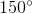each. What is the measure of the fifth angle?

This pentagon cannot exist.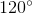This pentagon cannot exist.

Explanation:

The angles of a pentagon measure a total of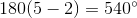. If we let the unknown angle measure be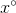, then from this information: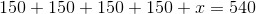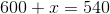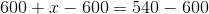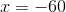Since an angle measure cannot be negative, this pentagon cannot exist.

### Example Question #2 : How To Find An Angle In A Pentagon

The measures of the angles of a pentagon are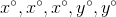. If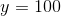, what is?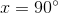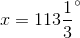This pentagon cannot exist.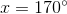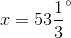Explanation:

The angles of a pentagon measure a total of. From the information, we know that: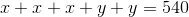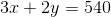If, then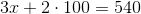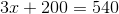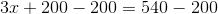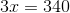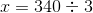### Example Question #3 : How To Find An Angle In A Pentagon

The measures of the angles of a pentagon are. If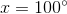, what is?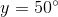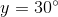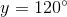This pentagon cannot exist.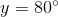Explanation:

The angles of a pentagon measure a total of. From the information, we know:If, then the above becomes: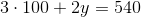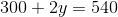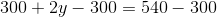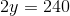### Example Question #121 : Isee Upper Level (Grades 9 12) Mathematics Achievement

Solve for: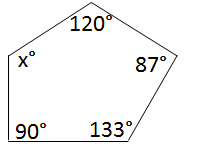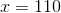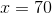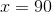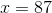Explanation:

The sum of the interior angles of a pentagon can be determined by the following equation, whereis the number of sides: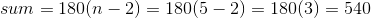Therefore: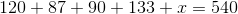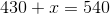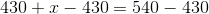### All ISEE Upper Level Math Resources